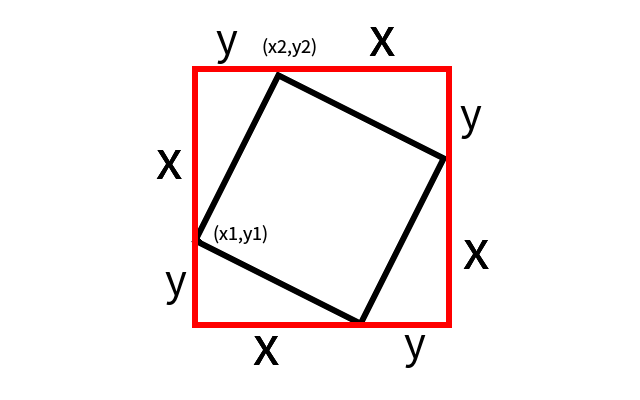# 2. 题解#include <cstdio>
#include <set>
#define MKP make_pair
using namespace std;
typedef pair<int,int> PII;
int n,ans,x,y;
set<PII> SET;
int main()
{
while(scanf("%d",&n),n)
{
for(int i=1,a,b;i<=n;i++)
scanf("%d%d",&x[i],&y[i]),SET.insert(MKP(x[i],y[i]));
for(int i=1;i<=n;i++)
for(int j=1;j<=n;j++)
{
int x1=x[i],y1=y[i],x2=x[j],y2=y[j],dx,dy;
if(x1>=x2||y1>y2)continue;
dx=x2-x1,dy=y2-y1;
ans+=SET.count(MKP(x1+dy,y1-dx))&&SET.count(MKP(x2+dy,y2-dx));
}
printf("%d\n",ans);
ans=0,SET.clear();
}
return 0;
}